• 三种方式 enumerate、itemize、description环境 具体可以参考链接LaTeX 使用：itemize,enumerate,description 用法 缩进设置 这一部分可以参考itemize, enumerate枚举，编号使用及编号样式设计 ...
三种方式
enumerate、itemize、description环境
具体可以参考链接LaTeX 使用：itemize,enumerate,description 用法
缩进设置
这一部分可以参考itemize, enumerate枚举，编号使用及编号样式设计
这里要说明一下，在这篇博客中，使用enumitem宏包设置enumerate环境下的缩进时，使用的以下代码：
\begin{enumerate}[fullwidth,itemindent=2em,label=(\arabic*)]

label=(\arabic*)这句话中的\arabic*代表的是序号，不要改。加了括号代表输出的形式类似于（1）这种，可以自己定义其他的形式。其他形式的选择可以参考官方文档enumitem。在这里我充当一个搬运工大概翻一下：
标题形式
主要有以下五种：
\alph
\Alph
\arabic
\roman
\Roman

还有另外一种比较特殊，需要使用到另外两个宏包：color和pifont
可以使用以下代码的形式
\begin{enumerate}[label=\protect\fcolorbox{blue}{yellow}{\protect\ding{\value*}}]

效果大概是这样的：展开全文• ## Latex公式编号问题

万次阅读 多人点赞 2019-12-25 21:37:43
目录对某个公式编号/不编号 在写文章时，我们会遇到各种各样的对公式编号的要求，...latex中给我们提供了很多编辑公式的方法，具体可在终端（命令提示符窗口）输入如下命令查看官方文档，这里我们做简单总结。 ...
目录对某个公式编号/不编号对某些公式整体编号较长公式拆行与编号
在写文章时，我们会遇到各种各样的对公式编号的要求，例如对某些公式标号而对另外一些公式不编号、对某些公式整体编号、对一个拆为几行的较长的公式的最后一行编号等。这篇文章总结了对上面三种情况的处理方法，后面遇到其他情况再回来补充。
对某个公式编号/不编号
latex中给我们提供了很多编辑公式的方法，具体可在终端（命令提示符窗口）输入如下命令查看官方文档，这里我们做简单总结。
texdoc lshort			%英文版
texdoc lshort-zh		%中文版
虽然latex提供了如下的方法用于输入单个公式，但是并不推荐使用这种方法。
$x^2+y^2=r^2$                   %行内公式
$$x^2+y^2=r^2$$                 %行间公式
单独成行的行间公式通常由equation环境包裹，equation环境自动为公式生成一个编号，这个编号可以通过 “\label” 和 “\ref” 生成交叉引用，还可以通过amsmath的 “\eqref” 命令为引用自动加上圆括号（若要使用 “\eqref” 命令，则须在导言区加上amsmath宏包），若引入了amsmath宏包，还可以使用 “\tag” 命令手动修改公式编号，或使用 “\notag” 命令取消为公式编号（若不想为公式编号，可直接使用equation*环境）。以下面的例子说明：
代码：
\documentclass{article}
\usepackage{amsmath}
\begin{document}
The Pythagorean theorem is:
\begin{equation}
a^2 + b^2 = c^2 \label{pythagorean}
\end{equation}
Equation \eqref{pythagorean} is
called ‘Gougu theorem’ in Chinese.
It’s wrong to say
\begin{equation}
1+1=3 \tag{dumb}
\end{equation}
or
\begin{equation}
1+1=4 \notag
\end{equation}
\end{document} 
编译结果如下图所示（因为存在交叉引用，故需要编译两次）：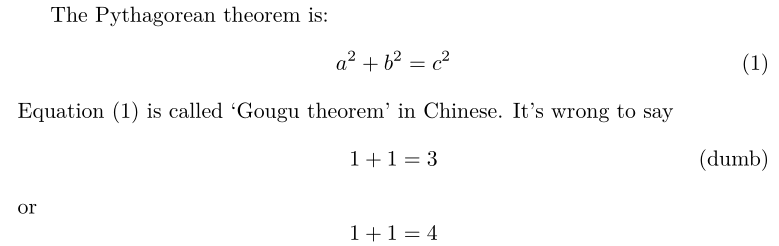注：

数学模式中输入的空格将被忽略，需要人为引入间距时，使用 “\quad”、 "\qquad"等命令。
不允许有空行（分段）。行间公式也无法使用 ‘’\" 命令换行，排版多行公式的办法下面小节中给出。
所有的字母被当做数学公式中的变量处理，字母间距与文本模式不一致，也无法生成单词之间的空格。如果想在数学公式中输入正体的文本，可用amsmath提供的 “\text” 命令，举例如下：

\documentclass{article}
\usepackage{amsmath}
\begin{document}
$x^{2} \geq 0 \qquad \text{for all}\quad x\in R$
\end{document}
效果如下图所示：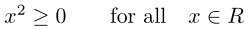对某些公式整体编号
某些时候要求我们对某几个公式整体进行编号，例如下面的例子：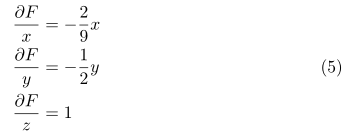方法一代码：
\begin{equation}
\begin{split}
\frac{\partial F}{x}&=-\frac{2}{9}x \\
\frac{\partial F}{y}&=-\frac{1}{2}y \\
\frac{\partial F}{z}&=1
\end{split}
\end{equation}
方法二代码（此方法依赖amsmath宏包）：
\begin{equation}
\begin{aligned}
\frac{\partial F}{x}&=-\frac{2}{9}x \\
\frac{\partial F}{y}&=-\frac{1}{2}y \\
\frac{\partial F}{z}&=1
\end{aligned}
\end{equation}
如果我们不需要等号对齐，而是居中对齐即可，那么用上述代码默认是又对其，下面的代码可使多行公式居中对齐。
\begin{gather}
\frac{\partial F}{x}=-\frac{2}{9}x \\
\frac{\partial F}{y}=-\frac{1}{2}y \\
\frac{\partial F}{z}=1
\end{gather}

较长公式拆行与编号
当我们遇到较长的公式，无法排版在一行时，就要求我们在适当的位置对公式进行拆分，并依照某种方式对齐，这里最常用的是align环境，它将公式用 “&” 隔为两部分并对齐，分隔符通常放在等号左边。align环境会给每行公式都编号，可使用 “\notag” 命令去掉某行编号，下面我们给出几个例子。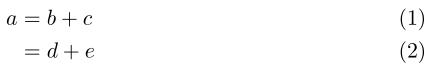代码：
\begin{align}
a&=b+c \\
&=d+e
\end{align}
若要取消对其中某行公式编号，则只需在相应的行使用 “\notag” 命令即可，如下：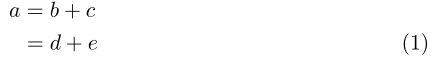代码：
\begin{align}
a&=b+c \notag \\
&=d+e
\end{align}
按加号对齐（为了对齐加号，我们将分隔符放在等号右边，这时需要给等号后添加一对括号 " {}"  以产生正常的）间距：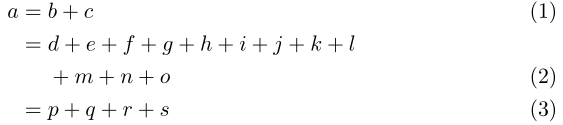代码：
\begin{align}
a ={} & b + c \\
={} & d + e + f + g + h + i
+ j + k + l \notag \\
& + m + n + o \\
={} & p + q + r + s
\end{align}


展开全文• latex 中，使用align时，发现换行后每一行都有编号。可以通过notag设置。 步骤 \begin{align} E_{1st}(M(\theta,\beta,\gamma);S) &= E_{\text{dis}} + \lambda_{\text{shape}}E_{\text{shape}} + \lambda_{\...
综述
latex 中，使用align时，发现换行后每一行都有编号。可以通过notag设置。
步骤
\begin{align}
E_{1st}(M(\theta,\beta,\gamma);S) &= E_{\text{dis}} + \lambda_{\text{shape}}E_{\text{shape}} + \lambda_{\text{p-prior}}E_{\text{p-prior}} \notag\\
&\begin{array}{l@{\quad}l@{}l@{\quad}l}
& + \lambda_{\text{s-prior}}E_{\text{s-prior}} \label{Zb}\\
\end{array}.
\end{align}



展开全文公式 换行
• ## Latex插入编号

千次阅读 2016-10-19 13:34:54
\item latex \item latex \item latx \end{itemize}\begin{itemize} \item[-] latex \item[-] latex \item[-] latx \end{itemize} 这样子就是用-取代实心点，也可以换成其他的字符{enumerate}命令\be
{itemize}命令

\begin{itemize}
\item latex
\item latex
\item latx
\end{itemize}

\begin{itemize}
\item[-] latex
\item[-] latex
\item[-] latx
\end{itemize}


这样子就是用-取代实心点，也可以换成其他的字符

{enumerate}命令

\begin{enumerate}
\item latex
\item latex
\item latx
\end{enumerate}


该命令可以实现自动标号
展开全文• latex 中的 \tag{} 是个可以给公式指定编号的, 这里涉及到 latex 公式编号的取消与替代, 可以参考 latex:公式的序号 做为更进一步的了解. 但是要注意, 虽然可以使用自己定义的编号, 但是可以看出, 公式的计数器是...
• \nonumber可以去除编号 或\tag{}修改 \begin{equation*} \begin{aligned} % requires amsmath; align* for no eq. number \emph{y}_{\emph{i+1}} & =\emph{terminal}(\emph{y}_{\emph{i}},\alpha(\emph{y}_{\...
• ## Latex项目编号

千次阅读 2015-02-05 15:30:09
生成黑点项目编号 \begin{itemize} \item \textbf{Applies widely}\\ This system can be used for many types of airplanes, and it also solves the interference during the procedure of the boarding ...
• 在Markdown中使用LaTeX\LaTeXLATE​X公式时，加上\tag{...}标签可以生成对应的编号。 比如这个代码可以生成 $$x+y = z \tag{1.1}$$ 这样的带编号的公式。 比如这个代码可以生成 (1.1)x+y=zx+y = z \tag{1.1}x+y=z...Markdown
• ## Latex公式及编号

千次阅读 2017-04-07 09:31:14
Latex公式及编号 1、latex公式加编号 如果用eqnarray环境，只能将编号显示在上面或下面等式的后面，如： \begin{eqnarray} a+b = 1 \\ c+d = 2 \end{eqnarray} 编号显示在第二个等式的后面。   ...公式
• \begin{enumerate} \item [(1)] \item [(2)] \end{enumerate}
• ## Latex 公式指定编号

千次阅读 2020-05-31 21:28:06
为了在使用 Latex 时可以自己设置公式的序号，可以通过\tag {序号} 实现，比如： z=x+y(15) z = x + y (15) z=x+y(15) 使用下面的 Latex 代码： \begin{equation} z = x + y \tag {15} \end{equation}
• \Large{\textcircled{\small{1}}} 效果： 或 \textcircled{1} 效果： 或 \textcircled{\small{1}} 效果：
• LaTeX公式编号 问题： 如何让LaTeX公式按照章节编号？ 答案： 在导言区加入如下语句。 \usepackage{amsmath} \numberwithin{equation}{section}
• ## LaTeX无图片编号

千次阅读 2017-06-12 23:47:51
编译LaTeX文件的时候发现\ref的地方没有编号，是”?”用作占位符。尝试之后发现是\label位置的关系，应该放在\caption后面。下面是正确的位置：\begin{figure} \centering \includegraphics[width=\textwidth]{...图片 标签
• 子公式编号，并且后面要对整个公式交叉引用的办法： 多个子公式逐行排列（无需大括号） \begin{subequations} \begin{align} x + y = 1 \\ x - y = 0 \end{align} \label{eq} \end{subequations} 子公式会...
• latex 表格引用编号How to compress lists of consecutive citation numbers to one number range in Latex? 如何在Latex中将连续引用数字列表压缩到一个数字范围？ For example, change 例如改变 , , [16...
• 文章目录Typora中LaTex公式手动编号 Typora中LaTex公式手动编号 最近在用Typora练习写笔记，有些地方需要用到公式，就用了里面的公式块功能。使用过程中发现使用使用eqution时不会自动编号，找了一下后找到个小方法...Typora...